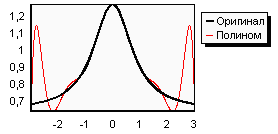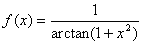# Polynomial interpolation

Polynomial interpolation is the most known one-dimensional interpolation method. Its advantages lies in its simplicity of realization and the good quality of interpolants obtained from it. However, it has several disadvantages (some of them will be considered later) and is lately hard-pressed by alternative interpolation methods: splines and rational functions. But, in spite of that, polynomial interpolation is still one of the main tools of numerical analysis.

# Theory

## Barycentric representation of polynomial

It is known that any rational function may be represented in barycentric form:The same formula may be used to represent polynomial, which is a special case of a rational function. Such representation have following properties: a) it is more stable than the power basis, b) after barycentric representation is built it takes only O(N) time to interpolate (instead of O(N 2) for Neville-type algorithm), c) in several important cases (equidistant or Chebyshev grids) barycentric model can be built in O(N) time instead of O(N 2).

## Choosing nodesThe interpolation accuracy depends on the interpolation nodes. The equidistant grid, despite its simplicity and convenience, should not be used for interpolation because of two related reasons.

To understand the first reason, let's consider the function graph. Here are the function(black) and the interpolation polynomial constructed by using 11 points of the equidistant grid (red). We can see that the interpolation accuracy is good in the center. The closer to the edges the less accurate the interpolation becomes. Although the polynomial passes through all the points, between them it deviates wildly from the function. We could expect that the interpolation error will decrease when we take more points. But in practice, the larger n we take, the wilder the deviation of the interpolation polynomial becomes from the function (near the edges). If the number of points goes to infinity, the interpolation error will tend towards infinity too.

It is proved that there is a class of functions which cannot be interpolated by the polynomial on an equidistant grid. These functions have poles on the complex plane in the neighborhood of the interpolation interval (in particular, the function mentioned above has poles in points x = +i and x = -i). It should be understood that this effect is not a bug in the algorithm and not a consequence of the floating point errors. It is a fundamental property of an interpolation polynomial.

The second reason not to use the equidistant grid is associated with the first one. When using the equidistant grid, floating point errors could be accumulated and cause low interpolation quality. The reason is even if the function to be interpolated is a "good function" (i.e. hasn't any poles in the neighborhood of the interpolation interval), floating point errors disfigure its graph. These small distortions can make this function look like a "bad function", which will increase an error dramatically.

This problem has two solutions. If we cannot back away from the equidistant grid, we can use cubic splines or rational functions. If we can choose the nodes, we can interpolate the function using Chebyshev nodes (the node arrangement is presented later). On this grid in the overwhelming majority of cases the interpolation error decreases when increasing the number of points (specifically, it is true for all smooth functions). The floating point errors are significantly lower too.

# Polynomial interpolation

## Functions

You can use following functions to build barycentryc representation of the polynomial interpolant:

• polynomialbuild - to build interpolant using function values sampled on an arbitrary grid using algorithm with O(N 2) complexity (however, interpolation itself will have O(N) complexity).
• polynomialbuildeqdist - to build interpolant using function values sampled on an equidistant grid (O(N) complexity).
• polynomialbuildcheb1 - to build interpolant using function values sampled on an Chebyshev-I grid (O(N) complexity).
• polynomialbuildcheb2 - to build interpolant using function values sampled on an Chebyshev-II grid (O(N) complexity).

Functions mentioned above return barycentricinterpolant structure as result. This structure can be used with following functions from ratint subpackage:

• barycentriccalc, which calculates interpolant value at given point
• barycentricdiff1 and barycentricdiff2, which calculate interpolant value and derivatives at given point
• barycentriclintransx, which makes linear change of variables x=a·t+b
• barycentriclintransy, which applies linear transformation to the polynomial P(x)=a·P(x)+b

Following functions are used to switch between barycentric representation and other representations:

• polynomialbar2pow - transforms polynomial from barycentric from barycentric to power basis (use it if you need polynomial coefficients)
• polynomialpow2bar - transforms polynomial from power basis to barycentric form
• polynomialbar2cheb - transforms polynomial from barycentric form to Chebyshev basis
• polynomialcheb2bar - transforms polynomial from Chebyshev basis to barycentric form

Finally, following functions can be used for quick - O(N) - evaluation of interpolating polynomial on one of the special grids without creation of barycentric model:

• polynomialcalceqdist - for a equidistant grid
• polynomialcalccheb1 - for a Chebyshev-I grid
• polynomialcalccheb2 - for a Chebyshev-II grid

## Examples

ALGLIB Reference Manual includes following examples on polynomial interpolation:

• polint_d_calcdiff - shows how to do polynomial interpolation using barycentric representation
• polint_d_conv - shows how to convert between barycentric representation and power (Chebyshev) basis
• polint_d_spec - shows how to work with special grids

# Polynomial curve fitting

ALGLIB package supports polynomial curve fitting, either unconstrained (polynomialfit function) or constrained (polynomialfitwc function). Arbitrary number of constraints on function value - f(xc)=yc - or its derivative - df(xc)/dx=yc - is supported. This functionality is described in more details in the corresponding chapter of ALGLIB User Guide.

ALGLIB Project offers you two editions of ALGLIB:

ALGLIB Free Edition:delivered for freeoffers full set of numerical functionalityextensive algorithmic optimizationsno low level optimizationsnon-commercial license

ALGLIB Commercial Edition:flexible pricingoffers full set of numerical functionalityextensive algorithmic optimizationshigh performance (SMP, SIMD)commercial license with support plan

## ALGLIB 3.16.0 for C++C++ library.
Delivered with sources.
Monolithic design.
Extreme portability.
Editions:   FREE   COMMERCIAL

## ALGLIB 3.16.0 for C#C# library with native kernels.
Delivered with sources.
VB.NET and IronPython wrappers.
Extreme portability.
Editions:   FREE   COMMERCIAL

## ALGLIB 3.16.0 for DelphiDelphi wrapper around C core.
Delivered as precompiled binary.
Compatible with FreePascal.
Editions:   FREE   COMMERCIAL

## ALGLIB 3.16.0 for CPythonCPython wrapper around C core.
Delivered as precompiled binary.
Editions:   FREE   COMMERCIAL